[Contents]

Positive and Negative
John Denker

The objective here is to explain the mathematical idea of negative. This includes negative numbers, but actually the idea of negative applies to lots of things, not just numbers. There are actually at least three distinct but interrelated ideas involved.

Among other things, we explain what people mean when they say “the negative of a negative is a positive”. We reinterpret that to mean “the opposite of the opposite is the original thing”.

Along the way we will learn a few things about vectors. (The idea of “opposite” applies quite generally, even to things that aren’t vectors, but the vectors make the situation easier to visualize.)

## 1  Basic Concepts and Terminology

The terminology surrounding subtraction and negation is a disaster. I’ve seen students who understand the concepts but can’t cope with the confusing terminology.

### 1.1  Subtraction, Negation/Opposition, and Subzero

For starters, we must distinguish between

1. subtraction, and
2. negation aka opposition, and
3. subzero numbers

In particular:

1. Given two numbers A and B, you can subtract them. For example: 5 minus 3 makes 2.
2. Given a single number B, you can negate it: the negative of 5 is negative 5, which we can write as [−5]. Similarly, given a vector V, we say that [−V] is the opposite of V.
3. The number [−3] is subzero, i.e. less than zero.

These are three profoundly different concepts ... but alas they are represented by the same symbol. The so-called “minus sign” is indistinguishable from the so-called “negative sign”.

Subzero numbers are customarily called «negative» numbers, but this is very misleading.

 For any B whatsoever, if B was subzero to begin with, then the opposite of B is greater than zero. The concept is simple. Unfortunately, the opposite of B is customarily called the negative of B, and written with a negative sign, namely [−B]. The terminology is confusing.

 Let’s be clear: When B is subzero, the so-called negative of B is not a negative number.

Sometimes it helps to distinguish negation from subtraction by writing the negative sign above the number. For example, negative three can be written as 3. If you’re writing in some medium (such as email) where it is not convenient to write a line over the number, you can use brackets to communicate the same idea: Negative three can be written as [−3]. The brackets emphasize that the negative sign is very tightly bound to thing that is being negated. It a negative sign, not a subtraction sign. For example, [−2]•[−3] means negative two times negative three; it is a multiplication problem pure and simple; there is no subtraction involved.

Negation is of course related to subtraction.

• Negation can be calculated based on subtraction (assuming you know what zero is): 3 := 0 − 3.
• Conversely, subtraction can be calculated based on negation (assuming you know about addition). 5 − 3 := 5 + 3. That’s pronounced five minus three is made by five plus negative three.
• The official mathematical notion of negation is defined in terms of addition and the concept of zero: If x + 3 = 0 then x is what we call negative 3, denoted 3 (or more conventionally [−3]).

### 1.2  Remarks

1. It is conventional and reasonable to pronounce (AB) as “A minus B”. This is pretty much the only reasonable pronunciation. Minus means subtraction. So far so good.
2. Alas, for [−B] there are two different pronunciations in common use:

 There are good reasons to pronounce [−B] as “negative B”. Everybody will understand you if you say that. It is all-too-common for people to pronounce [−B] as «minus» B, even though that conflicts with the previous definition. In this context minus means negation, not subtraction.

3. For subzero numbers, there are two different pronunciations, both of which are ambiguous and deceptive.

It is good for the teacher to distinguish between subtraction, negation, and subzero – even if such niceties are often lost on the students. Yes, the teacher should speak precisely, but it won’t pay off unless/until the students learn to hear precisely.

Along the same lines, it helps to talk about "subzero numbers" (rather than «negative numbers») when the number is less than zero. This allows us to say things like negative B is not necessarily a subzero number. If B was subzero to begin with, then [−B] is a positive number.

If we are dealing with things like vectors rather than numbers, only two of the three ideas enumerated in section 1.1 survive. Subtraction and negation are well defined for vectors, but there is no such thing as «less than zero» for vectors (in any vector space with more than one dimension).

The situation is summarized in figure 1. The columns represent the three concepts: Subtraction, Negation, and checking whether something is Subzero. The rows represent the various possible terms.Figure 1: Snafu: Subtraction, Negative, and Subzero

The green, boldface entries on the diagonal are the most logical terms for each given concept. In each column, the underlined terms are the most common terms for that concept. You can see that in no case is the logical term reliably more common than the conflict-plagued terms. Did you think mathematicians are smart? This diagram proves that mathematicians are just as foolish as everybody else.

The situation is somewhat better if we use mathematical symbols, as shown in the last line of the diagram. The “−” sign is ambiguous, meaning either subtraction or negation, but we can repair that by putting the negative sign in square brackets. With experience you can learn to distinguish the negative sign from the minus sign based on context, based on some tricky rules, but when in doubt, stick in plenty of brackets and parentheses.

Keep in mind that for vectors, the rightmost column does not exist at all. There are no subzero vectors.

### 1.3  Double Negative

Yet another fiasco arises when students hear that «two negatives make a positive». That’s not the right way to say it. Instead:

• A subzero plus a subzero just gets farther below zero. Example: (−2) + (−3) = −5.
• A subzero number times a subzero number goes in the opposite direction, i.e. above zero, as discussed in section 2. Example: (−2) × (−3) = +6.
• If we’re talking about vectors, there’s no such thing as a subzero vector ... but there is such a thing as one vector being the negative of another.
• The negative of a negative gives you back what you started with, as discussed in section 2. Example: −[−3] = +3. This works fine for lots of things, including numbers, vectors, et cetera.

## 2  An Introductory Example: 180 Degree Turns

Suppose you start out heading roughly towards the northwest, with velocity A, as shown in figure 2. Then you make a 180 turn. Now you are going in the opposite direction, corresponding to velocity B in the diagram. A little while later you make another 180 turn. Now you are once again going roughly towards the northwest, corresponding to velocity C in the diagram.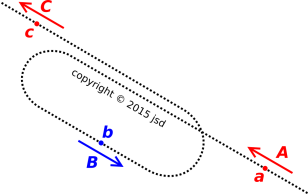Figure 2: Two 180-Degree Turns

Let’s be clear: If we pay attention only to direction, then B is the opposite of A, and C is the opposite of B. That means C is the same as A. We can make the following generalization: The opposite of the opposite is the same as the original thing.

## 3  Vectors

Sometimes it’s easier to learn two things together than to learn either one separately. In that spirit, let’s talk about vectors.

We define a vector to be something with direction and magnitude. Roughly speaking, you can visualize a vector as an arrow with a tail and a tip; the length of the arrow represents the vector’s magnitude, and the orientation of the arrow represents the vector’s direction. (For a more thorough discussion of what a vector is, see reference 1.)

The general idea of vector applies in any number of dimensions from 1 on up. However, 1-dimensional vectors are a little bit screwy. Most of what we say here is true for all vectors, but a few things are restricted to 2 dimensions and higher. These restricted statements are marked with the § symbol.

Vectors can be rotated§. Rotation changes the direction, but not the magnitude of the vector.

• Example: Velocity is a vector. Going 20 mph to the north is different from going 20 mph to the west, so it makes sense to say that velocity has magnitude and direction. The velocities in figure 2 are in this category.
• Example: Force is a vector. A push to the north is different from a push to the west, so it makes sense to say that force has magnitude and direction.
• Terminology: Something that has no direction is called a scalar.
• Counterexample: Temperature is a scalar, not a vector. It has magnitude but not direction. If you rotate something, its temperature does not change.
• Counterexample: Pressure is a scalar, not a vector. It has magnitude but not direction. Given a balloon with some pressurized gas inside, if you rotate the balloon, the pressure does not change.
• Counterexample: Plain old counting numbers are scalars. If you start with six carrots and rotate them, you still have six carrots. Six is a scalar.

In figure 2 vector A and vector C are equal. That’s because they have the same magnitude and direction. (They sit at different locations, but that’s irrelevant. Vectors are considered equal if they have the same direction and magnitude.)

A vector is not a number. In 1 dimension, the distinction between numbers and vectors is somewhat blurry, but in 2 dimensions or higher, you cannot even pretend that a vector is a number. Just as you cannot compare apples and oranges, you cannot§ compare vectors and numbers. In particular, you cannot§ say that a given vector is positive or negative, because that would require comparing the vector to zero. For details on this, see section 5.

I define higher math to be anything beyond arithmetic. Arithmetic deals with numbers. Here we are dealing with vectors, which are definitely§ not numbers. So we are doing higher math.

## 4  Multiplying Vectors by Scalars

By way of analogy, a carrot is not a number, but we know what it means to speak of six carrots. That is equivalent to multiplying carrot by six. A non-numerical object can be multiplied by a number, i.e. multiplied by a scalar.

So it is with vectors. In figure 3, we start with vector A. (It is the same vector A that we encountered in figure 2.) If we multiply A by 2, we get 2A, which has twice the magnitude of A, in the same direction. Similarly 3A has three times the magnitude of A, in the same direction.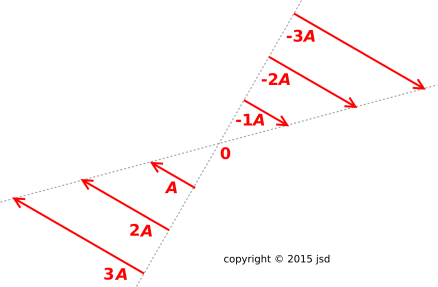Figure 3: Multiples of a Vector

Note that the expression 3A is synonymous with 3 times A, just as 6 carrots is synonymous with 6 times carrot. We could write 3A as 3×A or 3·A or 3*A or even 3∧A, but people usually just omit the multiplication operator. On the occasions when it is helpful, we can easily stick it back in (for instance in figure 4 and equation 3.)

Let’s keep going. We have considered 3A, 2A and 1A (which is another name for A). Next comes 0A, but let’s not worry about that. Then comes [−1]A. That has the same magnitude as A, but in the opposite direction. Similarly [−2]A has twice the magnitude of A, in the opposite direction.

Note that [−1]A is a perfectly good vector. In fact it is identical to the B vector in figure 2. It is not a “negative” vector; as mentioned in section 3 and especially section 5, there is no such thing§ as positive or negative vectors. You can say that B is the opposite of A, but A was abstract and arbitrary to begin with. You could equally well say that A is the opposite of B:

 A = −B B = −A
(1)

Note that [−A] means exactly the same thing as [−1]A, for the same reason that [−1] times 3 is [−3].

Since B is just another name for [−A], we can rewrite equation 1 as

 A = −[−A] B = −[−B]
(2)

which is a mathematical way of expressing something we observed in section 2: A is the opposite of the opposite of A. Similarly B is the opposite of the opposite of B.

Note that 3A is a perfectly good vector. We can do whatever we want with it, including multiplying it by 2. As you can see in figure 4, when we do that, we get something with twice the magnitude of 3A, in the same direction. Unsurprisingly, it is also equal to 6A. That is, it has six times the magnitude of A, in the same direction.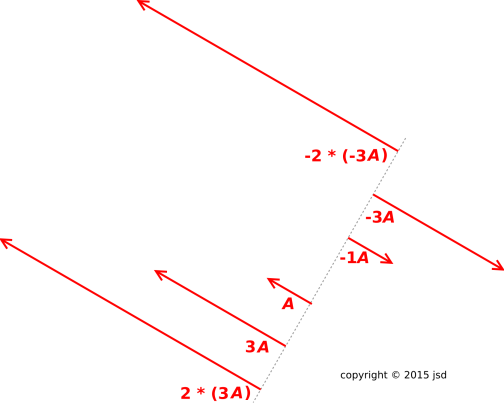Figure 4: Multiples of Multiples of a Vector

Last but not least, let’s consider what happens when we multiply [−3]A by [−2]. As you can see in figure 4, when we do that, we get something with twice the magnitude of [−3]A, in the opposite direction to [−3]A. That means it has the same direction as the original A vector, and six times the magnitude.

The idea behind figure 4 can be written in algebraic form as follows:

 2 * (3A) = 6A −2 * (−3A) = 6A
(3)

## 5  The Meaning of Negative

When we multiply a vector by a subzero number, it means to go in the opposite direction. It absolutely does not mean that the result goes in the «negative» direction. There never was any such thing as a negative direction§ anyway. Since B is equal to [−A], we can redraw the previous figure using multiples of B. This should make it even more obvious that [−2] * (3B) has to point in the opposite direction from B, i.e. in the same direction as A.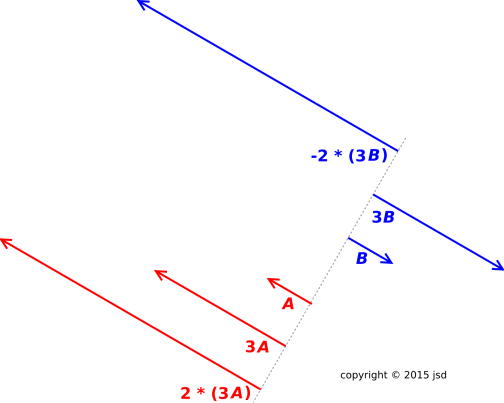Figure 5: Multiples of a Different Vector

Just as you cannot compare apples and oranges, you cannot§ compare vectors and numbers. In particular, you cannot§ say that a given vector is positive or negative, because that would require comparing the vector to zero.

Figure 6 may help explain what’s going on. Suppose your not-very-bright friend wants to define the W direction as «positive», and the Y direction as «negative». In the figure, these directions are opposite to each other, which seems like a promising sign.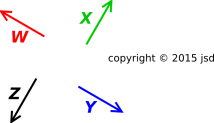Figure 6: Opposites, but no Negatives

However, a problem arises: The other vectors (X and Z) cannot be classified as positive or negative. They are opposite to each other, but neither aligned with nor opposite to the W and Y directions.

Another problem is that the choice of Y as the «negative» direction is at best arbitrary. You could dream up some other vector and choose it as your «negative» vector and it would work the same, i.e. not work at all.

## 6  Naturally Negative versus Relative Negative

There are some quantities in this world that can be positive, negative, or zero ... and everybody agrees on what the zero-level is. The canonical example is electric charge. The only problem with this example is that electric charge is an abstraction, which makes it hard to visualize correctly. (Any easy-to-visualize picture of electric charge is probably not completely correct.)

A great many quantities are naturally always positive or zero ... but subzero numbers can arise when we consider changes, or consider comparisons of one value relative to another.

Consider for example a game of checkers. At all times, the number of checkers is necessarily positive or zero. There is no such thing as a negative checker.

Each player starts out with 12 checkers, as shown in figure 7. Now suppose that some later point during the game, you have 7 pieces and the other player has 9, as shown in figure 8. That means that your status is [−5] relative to where you started ... and your standing is [−2] relative to the other player.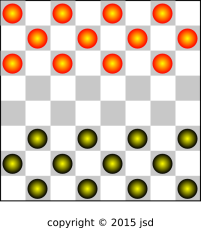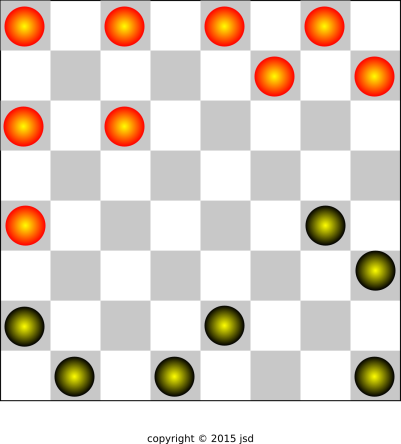Figure 7: Checkers – Initial Configuration Figure 8: Checkers – Later Configuration

Note that these subzero numbers are partly abstract. You can’t actually see the negative checkers. They are also partly arbitrary, insofar as the choice of reference is arbitrary. You could measure relative to what you started with, relative to the other player, or relative to something else entirely.

Another example in this category is topographic elevation. For many purposes, this is traditionally measured relative to mean sea level. The town of El Centro, California is below sea level. The elevation gives us a good example of something that’s negative and easy to visualize, as in figure 9. The tank is located at 32.77135N, 115.53895W and is rather large; you can judge the size by the handrails at the top of the dome. Ground level at this location is approximately 33 feet below sea level.Figure 9: Negative Elevation, Relative to Sea Level

Unlike (say) electric charge, the zero of elevation is somewhat arbitrary. There is no graven-in-stone commandment that requires us to measure elevation relative to sea level. If we measured relative to some lower reference, all elevations would be positive. Also note that the sea level itself is changing (relative to fixed benchmarks) due to climate change.

Here’s a similar example: In an airplane, the so-called true altitude is measured relative to mean sea level. Meanwhile, the so-called absolute altitude is measured relative to the local ground level. It is entirely possible to fly at a negative true altitude, for example when landing at Furnace Creek airport in Death Valley, California (field elevation 210 feet below sea level). Aircraft altimeters have no problem indicating negative altitudes.

Again the reference is somewhat arbitrary, as we can see from the fact that two different references are in use, and both are perfectly reasonable.

 True altitude is useful because changing altitude requires energy. In contrast, in level flight over uneven terrain, the absolute altitude can change wildly, but that is irrelevant to the energy budget.

 True altitude is convenient as a way of calculating the height of one airplane relative to another. On the other hand, when you are flying a few feet above the runway, on final approach, you care a very great deal about the absolute altitude.

An aircraft a few feet above the runway at Furnace Creek has a negative true altitude but a positive absolute altitude.

## 7  References

1.
John Denker,
“Introduction to Vectors”
www.av8n.com/physics/vector-intro.htm
[Contents]